## Diary — June 2021

#### June 1, 2021I tend to assume things will change slowly and continuously, but it's not always true. This is a dried lake bed in a Namibian desert.

#### June 4, 2021

I taught my last class at U.C Riverside! At least the last class I need to teach.

Then I celebrated by going out for a burrito with Lisa.

#### June 5, 2021

Welcome to the strange world of protocarnivorous plants.

There are at least 583 species of carnivorous plants, with about 3 new species discovered each year since 2000.

They all live in environments severely short of nitrogen — often acidic bogs. This is the force that pushes plants to eat animals. But how does it start?

The details of how carnivorous plants evolved must differ from case to case, since they use 5 different kinds of traps — and carnivory has evolved independently at least 9 times in plants!

But there are also about 300 species of 'protocarnivorous' plants, and we can study those.Some non-carnivorous plants have hairy leaves.

Insects can get caught in water on these leaves and die, releasing nutrients that the plant absorbs through its leaves. At first this is 'just an accident' — but in places where nitrogen is rare, it becomes important.

The plants naturally evolve to have hairier leaves, so they catch more insects. The leaves may start to secrete sticky stuff. Sometimes the plants benefit from the poop of insects who make their living eating other insects that get caught on their leaves!

A plant has become a full-blown carnivore when its leaves have glands that secrete sticky stuff and 'proteases' — enzymes that speed the decay of the insects that get caught on the leaves.

Eventually we can get plants whose leaves are covered with tentacles tipped with sticky droplets.

And the leaves of the Cape sundew actively bend to wrap around insects that land on it!

There are other strange cases. In Chile, the plant Puya chilensis has enormous prongs. Sheep sometimes get caught on them and die. The rotting corpse fertilizes the plant.

Is this plant protocarnivorous? Carnivorous? Nobody is sure.

For more, see:

#### June 6, 2021

On Twitter someone pointed out that some of the mechanisms that carnivorous plants use to trap insects are also used by plants to force insects to pollinate them! See:
This leads to some interesting problems, especially because carnivorous plants are also often pollinated by insects. Could plants that use pollination traps evolve toward being carnivorous? Or conversely, could carnivorous plants evolve toward using pollination traps? Both moves seem risky even if rather easy in terms of the structural changes required. Some of these issues are discussed here:
• Andreas Jürgens, Amber Sciligo, Taina Witt, Ashraf M. El-Sayed and D. Max Suckling, Pollinator-prey conflict in carnivorous plants, Biological Reviews 87 (2011), 602—615.
Most carnivorous plants utilize insects in two ways: the flowers attract insects as pollen vectors for sexual reproduction, and the leaves trap insects for nutrients. Feeding on insects has been explained as an adaptation to nutrient-poor soil, and carnivorous plants have been shown to benefit from insect capture through increased growth, earlier flowering and increased seed production. Most carnivorous plant species seem to benefit from insect pollination, although many species autonomously self-pollinate and some propagate vegetatively. However, assuming that outcross pollen is advantageous and is a more important determinant of reproductive success than the nutrients gained from prey, there should be a selective pressure on carnivorous plants not to feed on their potential pollen vectors. Therefore, it has been suggested that carnivorous plants are subject to a conflict, often called the pollinator-prey conflict (PPC). The conflict results from a trade-off of the benefits from feeding on potentially pollinating insects versus the need to use them as pollen vectors for sexual reproduction. In this review we analyze the conditions under which a PPC may occur, review the evidence for the existence of PPCs in carnivorous plants, and explore the mechanisms that may be in place to prevent or alleviate a PPC. With respect to the latter, we discuss how plant signals such as olfactory and visual cues may play a role in separating the functions of pollinator attraction and prey capture.

#### June 9, 2021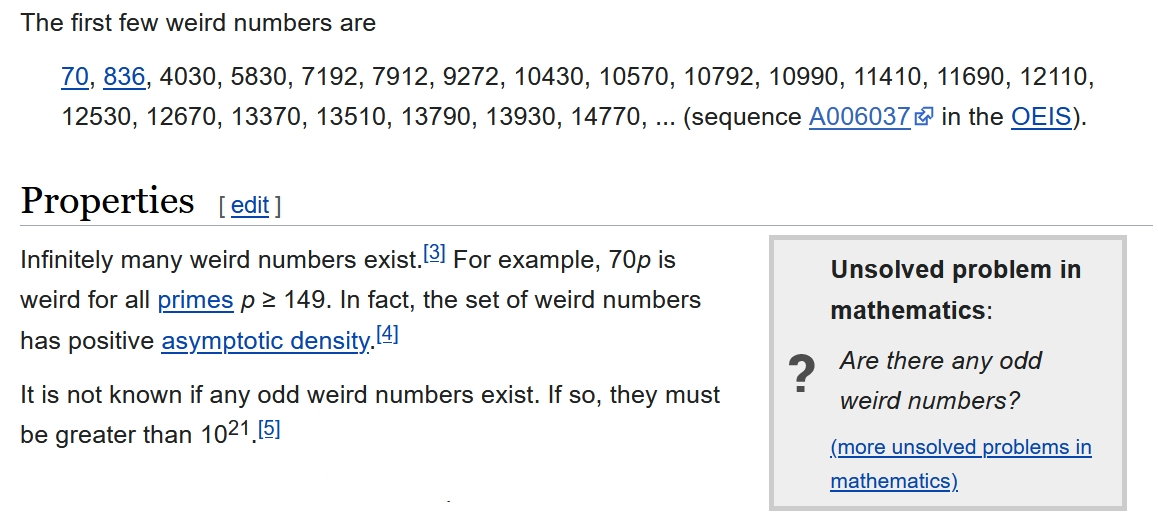This makes me smile. Mathematicians use a lot of funny language but I'd never seen this. So what's a 'weird number'?

A number $$n$$ is weird if it's abundant (the sum of its divisors including 1 but not itself is $$\gt n$$ but it fails to be semiperfect (no subset of those divisors sums to the number itself).

70 is the only weird number less than 100.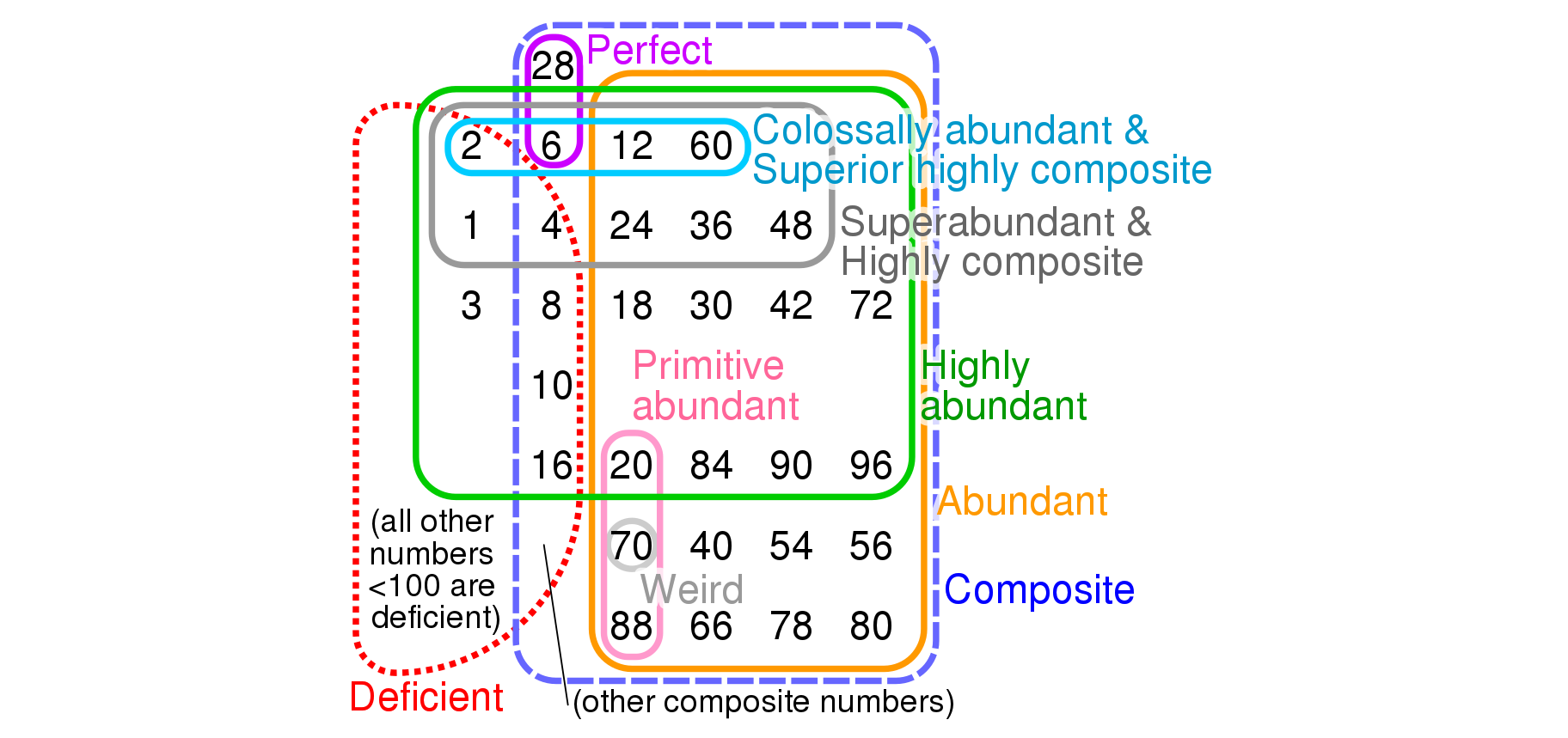The divisors of 70 other than itself are 1, 2, 5, 7, 10, 14, and 35. These sum to 74, which is $$\gt$$ 70, so 70 is abundant. But no subset of these sums to 70, so 70 fails to be semiperfect. So — some genius declared — 70 is weird.

These silly-sounding concepts are connected to some really hard mathematics. A number is perfect if it equals the sum of its divisors other than itself. The oldest unsolved math problem I know is this: are there infinitely many perfect numbers?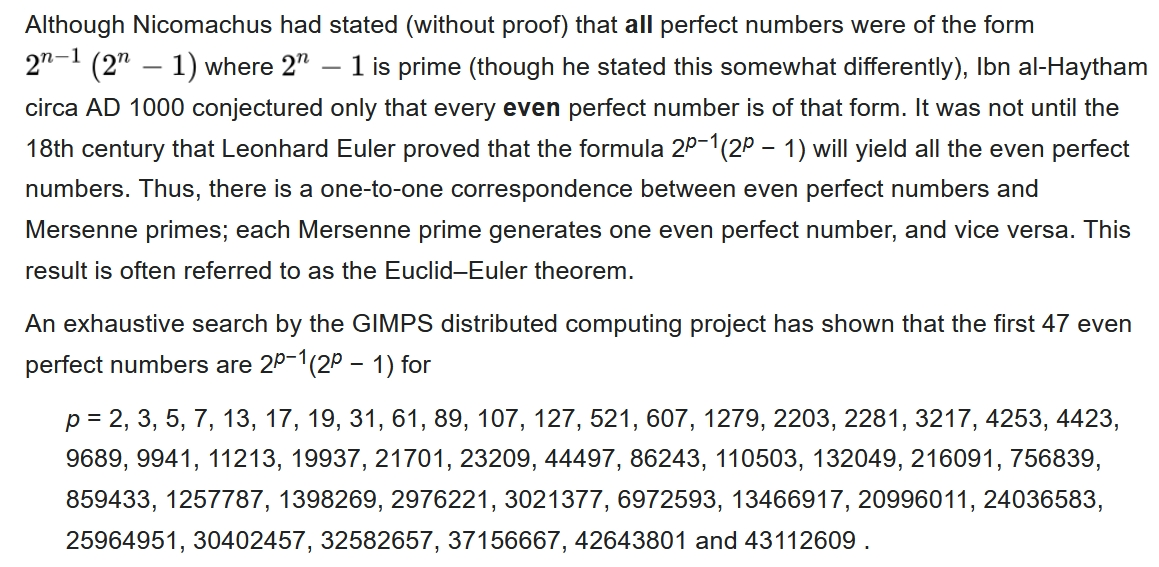Also, the Riemann Hypothesis is false iff you can find a "colossally abundant" number $$n \gt 5040$$ whose sum of divisors, divided by $$n \ln(\ln n)$$, is more than $$\exp(\gamma)$$.

The first few colossally abundant numbers are my friends. They go like this: $$2, 6, 12, 60, 120, 360, 2520, 5040, 55440, \dots$$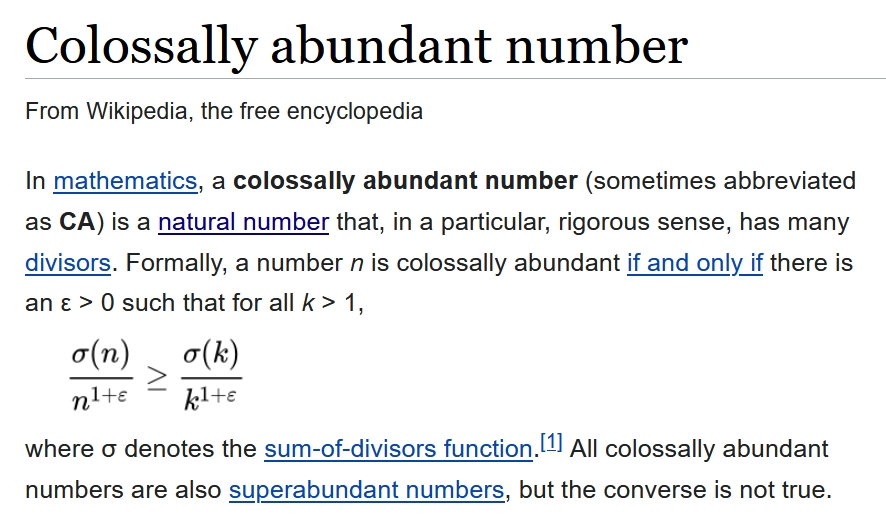I would never want to work on this kind of math. It's too hard, and — except for the Riemann Hypothesis, which is way too hard — not strongly connected to things I deeply care about. But it's fun to read about it now and then.

#### June 10, 2021Lines are very interesting. There are many ways to think about them. In algebraic geometry they study the 'affine line', called $$\mathbb{A}^1$$.

Let me explain that and say why it's a 'plethory'. I'll need to explain plethories too! I've been working on those lately.

The well-known 'real line', made of real numbers, is just one of many lines. In algebraic geometry, any field can be seen as a line. So they also talk about the 'complex line', meaning the complex numbers. Other people may call it a plane, but not them!

To handle all these lines simultaneously it's good to work with the integers, $$\mathbb{Z}$$. These form a more primitive sort of line. There's an obvious map from the integers to any field, so this line maps to all the other more fancy lines.

In algebraic geometry we study spaces by studying functions on these spaces. We study the real line using the commutative ring $$\mathbb{R}[x]$$ of polynomials with real coefficients.

We study the 'integer line' using the commutative ring $$\mathbb{Z}[x]$$ of polynomials with integer coefficients.

$$\mathbb{Z}[x]$$ is fundamental because it's the free commutative ring on one generator. This is what makes the 'integer line' so important.

Grothendieck said any commutative ring can be seen as the functions on a kind of space called an 'affine scheme'. Being a sneaky devil, he made this into a tautology: any commutative ring gives an affine scheme just by definition.

Study this and you'll get the joke here: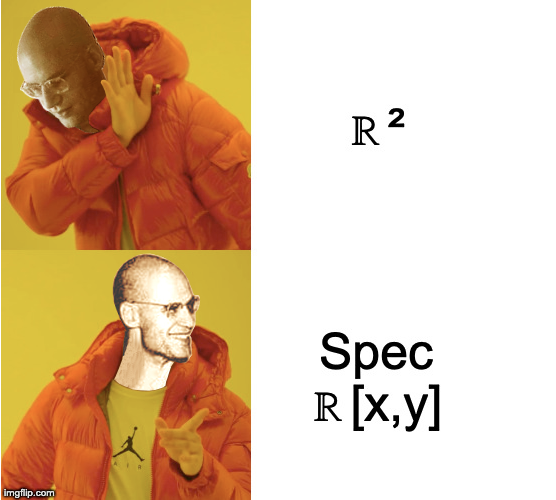A commutative ring in the opposite of the category of commutative rings is called a biring. Concretely this means that besides the usual ring operations, $$\mathbb{Z}[x]$$ also has 'co-operations' like this: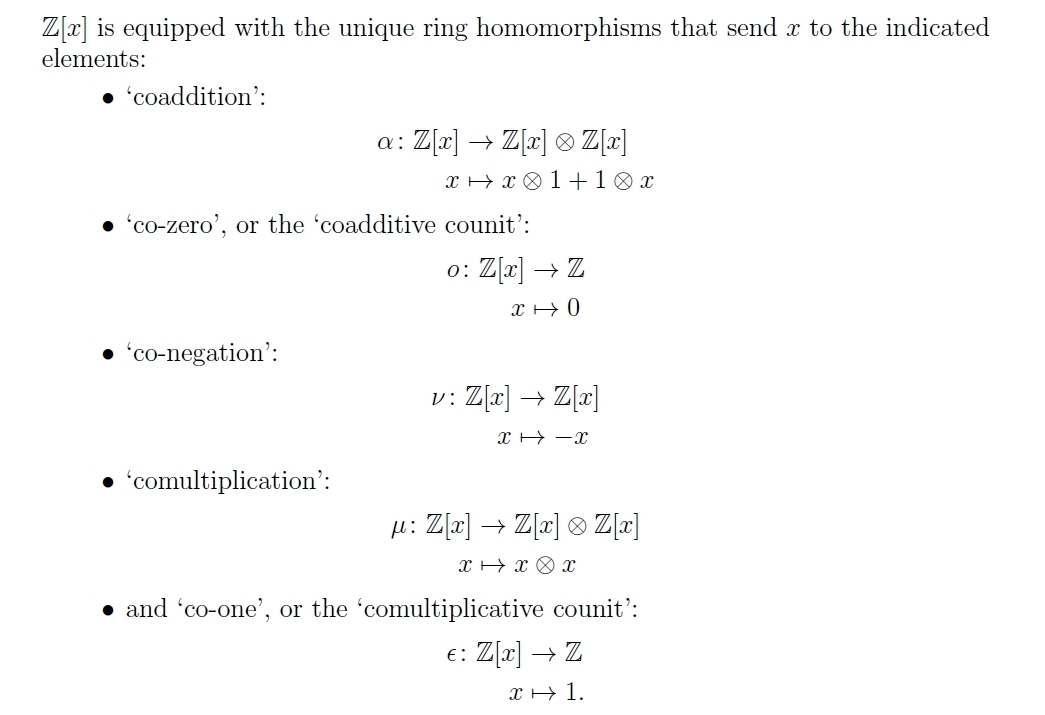But $$\mathbb{Z}[x]$$ is even better than a biring. It has even more structure, since we can also compose polynomial functions. So $$\mathbb{Z}[x]$$ is a plethory a monoid in the monoidal category of birings.

What does all this mean? It actually makes tons of sense. The affine line $$\mathbb{A}^1$$ is a commutative ring in the world of affine schemes — and also functions on this line act to map this line to itself, so we can compose them.

That's what makes it be a plethory.

The next step is to categorify all this stuff, and that's what Joe Moeller, Todd Trimble and I did! We showed that a famous category is actually a categorified version of the affine line.... and we proved it's a '2-plethory'. For a summary of this paper, and a link to the actual paper, go here:

#### June 12, 2021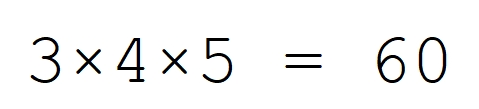I can't believe that I'm 60. Only yesterday I was in my prime.

Luckily next year I'll be in my prime again.

And 60 is a really cool number. It has a lot of divisors:

1, 2, 3, 4, 5, 6, 10, 12, 15, 20, 30, 60

which is probably why the Babylonians and the Mali Empire used base 60.

The buckyball has 60 carbon atoms:The buckyball, or geometricaly speaking the truncated icosahedron, also has 60 rotational symmetries. There's exactly one way to rotate it that carries any given vertex to any other vertex!

This makes it very different from the cube or icosahedron. It's a picture of its own symmetry group!More precisely: if we pick any carbon atom in the buckyball and call it $$1$$, the set of all its carbon atoms naturally becomes a group. Each carbon atom $$x$$ defines a rotational symmetry of the buckyball: namely, the unique rotation that carries $$1$$ to $$x$$.

So, we can multiply them. The carbon atoms in the buckyball thus form a 'torsor': roughly speaking, a group that's forgotten its identity.

Believe it or not, this affects the chemistry of the buckyball. Symmetry affects the spectral lines of a compound, and the fact that the symmetry group of the buckyball corresponds to its set of carbon atoms gives it special properties. You can read a lot more about this here:

• Fan R. Chung, Bertram Kostant, and Shlomo Sternberg, Groups and the buckyball, in Lie Theory and Geometry, Birkhäuser, Boston, 1994, pp. 97–126.
Part of the fun is that this 60-element group is isomorphic to $$\mathrm{A}_5$$, the group of all even permutations of the set $$\{1,2,3,4,5\}$$. It's also isomorphic to $$\mathrm{PSL}(2,5)$$, the group of transformations of the projective line over the field with 5 elements. This sets up a lot of interesting interplay between different branches of math, which the paper above explores.

Also, this group is the smallest nonabelian simple group!

So, I could easily spend the whole year studying the math connected to the buckyball and this 60-element group. And I won't be working at UCR anymore, so I could actually do this if I felt like it! (But I won't.)

When David Harden saw this, he wrote:

You said something about what's interesting about 60, but it's also worth noting that your age in months is now $$60 \times 12 = 6!$$ Exceptional objects are very fun, so let's relate this to $$\mathrm{A}_5$$.

$$\mathrm{A}_5$$ is, as you mentioned, the group of all even permutations on 5 points, and the rotational symmetry group of an icosahedron or dodecahedron. This is already pointing strongly in the direction of $$\mathrm{Aut}(\mathrm{S}_6)$$:

The rotational symmetry group of an icosahedron or dodecahedron can be seen as acting on 5 points (cubes in a dodecahedron, or octahedra in an icosahedron), but it has a much more self-evident action on 6 points: take the opposite pairs of vertices of an icosahedron/faces of a dodecahedron. This means $$\mathrm{A}_5$$ acts transitively on 6 points. (The description of this group as $$\mathrm{PSL}_2(5)$$, which you mentioned, also immediately highlights its 6-point action, since the projective line over $$\mathbb{Z}/5$$ contains 6 points.) Since 6 = (5-2)! is also the number of Sylow 5-subgroups of $$\mathrm{A}_5$$ this 6-point action can be described as the action of $$\mathrm{A}_5$$ by conjugation on its Sylow 5-subgroups. Any element of odd order in $$\mathrm{S}_5$$ is in $$\mathrm{A}_5$$, so the Sylow 5-subgroups of $$\mathrm{A}_5$$ are the Sylow 5-subgroups of $$\mathrm{S}_5$$. Then it becomes clear that this transitive action of $$\mathrm{A}_5$$ on 6 points can be extended to a transitive action of $$\mathrm{S}_5$$ on 6 points, since we just need to let odd permutations also permute the Sylow 5-subgroups of $$\mathrm{A}_5$$ by conjugation. Then comparing the action of $$\mathrm{S}_6$$ on the cosets of an intransitive, point-stabilizing $$\mathrm{S}_5$$ with its action on the cosets of a transitive $$\mathrm{S}_5$$ gives an outer automorphism of $$\mathrm{S}_6$$.

Backing up a bit, there's also, as I am pretty sure I've mentioned before to you, a way to construct the transitive $$\mathrm{S}_5$$ on 6 points using a Rubik's Cube.

Take two adjacent faces. Mixing up their face rotations in every possible way permutes the 6 vertices in the union of these faces according to a transitive $$\mathrm{S}_5$$ on 6 points. To verify this, it suffices to exhibit a partition of the 6 points (into 3 pairs) that has just 5 images under the action of the group generated by those two face rotations. So imagine looking at the cube so that only those two faces are in front of you. Two of the moved vertices are in the intersection of the two faces — this is a pair. The other pairs are the vertices on the left and the vertices on the right. (So this partition can be said to look like an H, or maybe something like that with parentheses on the sides.)

But why stop there? It's also fun to smell the sporadic roses along the way. $$\mathrm{A}_5$$ can't act transitively on 11 points, since 60 is not a multiple of 11. But putting the 5-point and 6-point actions of $$\mathrm{A}_5$$ side by side gives a 2-orbit action of $$\mathrm{A}_5$$ on 11 points. Thinking of these points as $$\mathbb{Z}/11$$, it's possible to choose an 11-cycle that extends the $$\mathrm{A}_5$$ action to a $$\mathrm{PSL}_2(11)$$ action on 11 points.

$$\mathrm{PSL}_2(11)$$ can't contain a subgroup isomorphic to $$\mathrm{S}_5$$, since 660 isn't a multiple of 120. So what happens if we repeat this construction with $$\mathrm{S}_5$$ in place of $$\mathrm{A}_5$$?

We can't get $$\mathrm{PGL}_2(11)$$: $$\mathrm{PGL}_2(11)$$ contains elements of order 10, which can't square to permutations of cycle shape $$(5,5)$$ unless they are 10-cycles. But in the 2-orbit action of $$\mathrm{S}_5$$, transpositions act oddly on both orbits, so they act evenly on the 11 points. Therefore all permutations in this group are even, and 10-cycles can't be among them. That argument also makes it clear that a Sylow 11-subgroup normalizer in this $$\langle \mathrm{S}_5, \mathrm{C}_{11}\rangle$$ must have order 55. Then the order of the group is 55 times the number of Sylow 11-subgroups. This group has a subgroup isomorphic to $$\mathrm{S}_5$$, so its order is a multiple of 120. This means the number of Sylow 11-subgroups of the group is a multiple of $$120/\mathrm{gcd}(120,55) = 24$$. Also, the number of Sylow 11-subgroups of this group is $$\equiv 1 (\bmod 11)$$. Combining $$n \equiv 1 \bmod 11$$ and $$n \equiv 0 \bmod 24$$ gives $$n \equiv 144 \bmod 264$$. So the order of this group is $$\ge 55 \times 144 = 7920$$. In fact, this lower bound can be sharp, when the group so generated is isomorphic to the Mathieu group $$\mathrm{M}_{11}$$. (Having the elements of the 5-point orbit in those nonzero square positions in the 11-cycle may be sufficient to ensure that the group so generated is $$\mathrm{M}_{11}$$. I am not sure about this.)

Remembering that extending the $$\mathrm{A}_5$$ in this construction to $$\mathrm{S}_5$$ led to extending $$\mathrm{PSL}_2(11)$$ to $$\mathrm{M}_{11}$$ also serves as a way to see that $$\mathrm{M}_{11}$$ has subgroups isomorphic to $$\mathrm{PSL}_2(11)$$. Since $$[\mathrm{M}_{11}:\mathrm{PSL}_2(11)] = 12$$, this gives a 12-point transitive action of $$\mathrm{M}_{11}$$. In fact, this 12-point transitive action of $$\mathrm{M}_{11}$$ can be regarded as analogous to the 6-point transitive action of $$\mathrm{A}_5$$ or $$\mathrm{S}_5$$ in the preceding. Likewise, some 23-cycles having the points of the 11-orbit in the nonzero square positions enable that 23-point, 2-orbit copy of $$\mathrm{M}_{11}$$ to be extended to $$\mathrm{M}_{23}$$.

A more complete telling of the story would, of necessity, venture to $$\mathrm{M}_{12}$$ and $$\mathrm{M}_{24}$$. But I am a bit tired for that at the moment. Continue doing well in this next year of your life!

#### June 13, 2021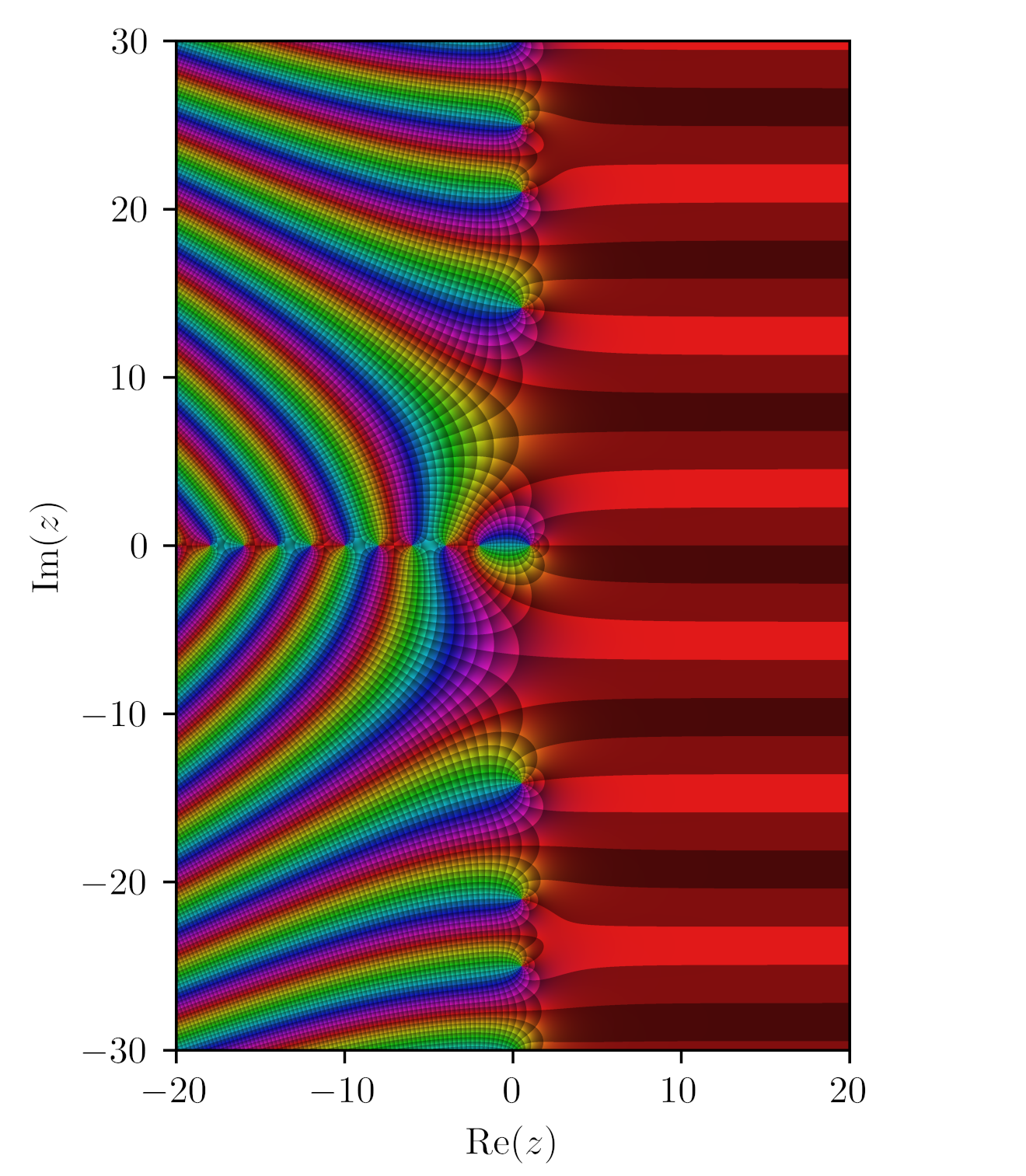A big question has always been: what do the nontrivial zeros of the Riemann zeta function mean? Until they correspond to some conceptually significant thing, it's hard to imagine proving they all lie on the line $$\mathrm{Re}(z) = 1/2$$.

In 1982, Pólya suggested that the nontrivial zeros of the Riemann zeta function should correspond to the eigenvalues of some interesting self-adjoint operator. Since then there's been a lot of work looking for this operator. This might give a physics-inspired proof of the Riemann Hypothesis:

Now Alain Connes and Caterina Consani claim to have an interpretation of Riemann zeta zeros as eigenvalues of an interesting operator. But — here's the catch — only the zeros that actually lie on the line $$\mathrm{Re}(z) = 1/2$$. This might still be interesting.

They start by defining the concept of a 'zeta-cycle', a circle whose circumference has a special property. The stuff here makes perfect sense to me except for the definition of the operator $$\Sigma_\mu$$, which I'd need to read more of the paper to understand.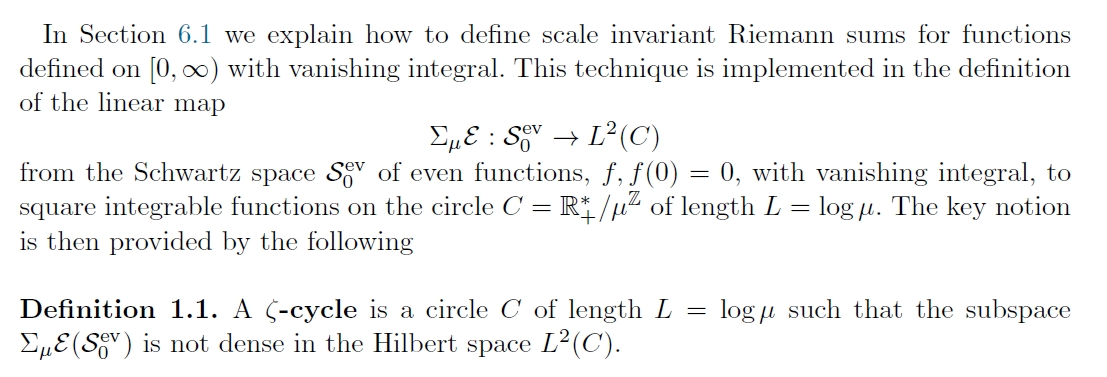Then, they claim to prove that the circumferences of these zeta-cycles are integer multiples of the imaginary parts of the Riemann zeta zeros that lie on the critical line $$\mathrm{Re}(z) = 1/2$$.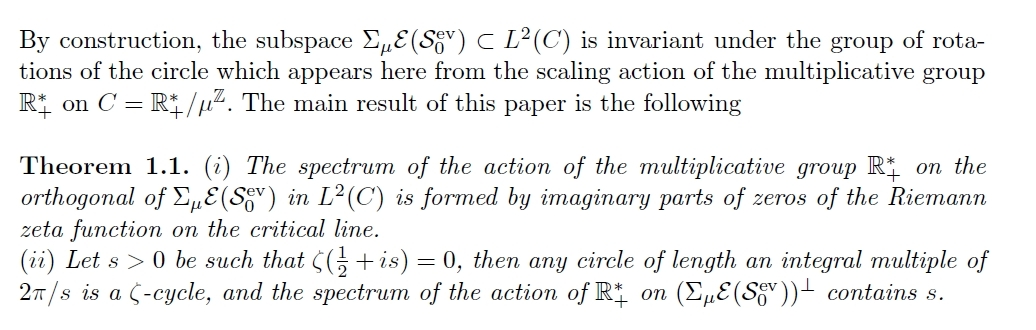Connes and Consani are very good mathematicians and they're not claiming to prove the Riemann Hypothesis so I give them a good chance of being right, though anyone can make a mistake. The big question would then be: how significant is this result?

They have been working on the Riemann Hypothesis since at least 2007 — maybe longer? — and have created quite an impressive pile of beautiful ideas. This paper is different, because some discoveries were made with the help of computer calculations.

So, while I don't understand this stuff very well, my interest is piqued. They seem to be "coming down to earth" with their research program, since now they're actually computing things, finding surprises — and they have a candidate for what a nontrivial Riemann zeta zero actually means.

Here is their paper:

#### June 15, 2021

This is a striking portrait of the "outsider genius" Jacob Obrecht:

Obrecht, ~1457–1505, was an important composer in the third generation of the Franco-Flemish school. While he was overshadowed by the superstar Josquin, I'm currently finding him more interesting — mainly on the basis of one long piece called Missa Maria zart.

Obrecht was very bold and experimental in his younger years. He would do wild stuff like play themes backwards, or take the notes in a melody, rearrange them in order of how long they were played, and use that as a new melody. Paraphrasing Wikipedia:

Combining modern and archaic elements, Obrecht's style is multi-dimensional. Perhaps more than those of the mature Josquin, the masses of Obrecht display a profound debt to the music of Johannes Ockeghem in the wide-arching melodies and long musical phrases that typify the latter's music. Obrecht's style is an example of the contrapuntal extravagance of the late 15th century. He often used a cantus firmus technique for his masses: sometimes he divided his source material up into short phrases; at other times he used retrograde (backwards) versions of complete melodies or melodic fragments. He once even extracted the component notes and ordered them by note value, long to short, constructing new melodic material from the reordered sequences of notes. Clearly to Obrecht there could not be too much variety, particularly during the musically exploratory period of his early twenties. He began to break free from conformity to formes fixes (standard forms) especially in his chansons (songs). However, he much preferred composing Masses, where he found greater freedom. Furthermore, his motets reveal a wide variety of moods and techniques.

But I haven't heard any of these far-out pieces yet. Instead, I've been wallowing in his masterpiece: Missa Maria zart, an hour-long mass he wrote one year before he died of the bubonic plague. Here is the Tallis Scholars version, with a score:

It's harmonically sweet: it seems to avoid the pungent leading-tones that Dufay or even Ockeghem lean on. It's highly non-repetitive: while the same themes get reused in endless variations, there's little if any exact repetition of anything that came before. And it's very homogeneous: nothing stands out very dramatically. So it's a bit like a beautiful large stone with all its rough edges smoothed down by water, that's hard to get a handle on. And I'm the sort of guy who finds this irresistibly attractive. After about a dozen listens, it reveals itself.

The booklet in the Tallis Scholars version, written by Peter Phillips, explains it better:

To describe Obrecht's Missa Maria zart ('Mass for gentle Mary') as a 'great work' is true in two respects. It is a masterpiece of sustained and largely abstract musical thought; and it is possibly the longest polyphonic setting of the Mass Ordinary ever written, over twice the length of the more standard examples by Palestrina and Josquin. How it was possible for Obrecht to conceive something so completely outside the normal experience of his time is one of the most fascinating riddles in Renaissance music.

Jacob Obrecht (1457/8–1505) was born in Ghent and died in Ferrara. If the place of death suggests that he was yet another Franco-Flemish composer who received his training in the Low Countries and made his living in Italy, this is inaccurate. For although Obrecht was probably the most admired living composer alongside Josquin des Prés, he consistently failed to find employment in the Italian Renaissance courts. The reason for this may have been that he could not sing well enough: musicians at that time were primarily required to perform, to which composing took second place. Instead he was engaged by churches in his native land — in Utrecht, Bergen op Zoom, Cambrai, Bruges and Antwerp — before he finally decided in 1504 to take the risk and go to the d'Este court in Ferrara. Within a few months of arriving there he had contracted the plague. He died as the leading representative of Northern polyphonic style, an idiom which his Missa Maria zart explores to the full.

This Mass has inevitably attracted a fair amount of attention. The most recent writer on the subject is Rob Wegman (Born for the Muses: The Life and Masses of Jacob Obrecht by Rob C Wegman (Oxford 1994) pp.322–330. Wegman, Op.cit., p.284, is referring to H Besseler's article 'Von Dufay bis Josquin, ein Literaturbericht', Zeitschrift für Musikwissenschaft, 11 (1928/9), p.18): 'Maria zart is the sphinx among Obrecht's Masses. It is vast. Even the sections in reduced scoring … are unusually extended. Two successive duos in the Gloria comprise over 100 bars, two successive trios in the Credo close to 120; the Benedictus alone stretches over more than 100 bars'; 'Maria zart has to be experienced as the whole, one-hour-long sound event that it is, and it will no doubt evoke different responses in each listener … one might say that the composer retreated into a sound world all his own'; 'Maria zart is perhaps the only Mass that truly conforms to Besseler's description of Obrecht as the outsider genius of the Josquin period.'

The special sound world of Maria zart was not in fact created by anything unusual in its choice of voices. Many four-part Masses of the later fifteenth century were written for a similar grouping: low soprano, as here, or high alto as the top part; two roughly equal tenor lines, one of them normally carrying the chant when it is quoted in long notes; and bass. The unusual element is to a certain extent the range of the voices — they are all required to sing at extremes of their registers and to make very wide leaps — but more importantly the actual detail of the writing: the protracted sequences against the long chant notes, the instrumental-like repetitions and imitations.

It is this detail which explains the sheer length of this Mass. At thirty-two bars the melody of Maria zart is already quite long as a paraphrase model (the Western Wind melody, for example, is twenty-two bars long) and it duly becomes longer when it is stated in very protracted note-lengths. This happens repeatedly in all the movements, the most substantial augmentation being times twelve (for example, 'Benedicimus te' and 'suscipe deprecationem nostram' in the Gloria; 'visibilium' and 'Et ascendit' in the Credo). But what ultimately makes the setting so extremely elaborate is Obrecht's technique of tirelessly playing with the many short phrases of this melody, quoting snippets of it in different voices against each other, constantly varying the extent of the augmentation even within a single statement, taking motifs from it which can then be turned into other melodies and sequences, stating the phrases in antiphony between different voices. By making a kaleidoscope of the melody in these ways he literally saturated all the voice-parts in all the sections with references to it. To identify them all would be a near impossible task. The only time that Maria zart is quoted in full from beginning to end without interruption, fittingly, is at the conclusion of the Mass, in the soprano part of the third Agnus Dei (though even here Obrecht several times introduced unscheduled octave leaps).

At the same time as constantly quoting from the Maria zart melody Obrecht developed some idiosyncratic ways of adorning it. Perhaps the first thing to strike the ear is that the texture of the music is remarkably homogeneous. There are none of the quick bursts of vocal virtuosity one may find in Ockeghem, or the equally quick bursts of triple-time metre in duple beloved of Dufay and others. The calmer, more consistent world of Josquin is suggested (though it is worth remembering that Josquin may well have learnt this technique in the first place from Obrecht). This sound is partly achieved by use of motifs, often derived from the tune, which keep the rhythmic stability of the original but go on to acquire a life of their own. Most famously these motifs become sequences — an Obrecht special — some of them with a dazzling number of repetitions (nine at 'miserere' in the middle of Agnus Dei I; six of the much more substantial phrase at 'qui ex Patre' in the Credo; nine in the soprano part alone at 'Benedicimus te' in the Gloria. This number is greatly increased by imitation in the other non-chant parts). Perhaps this method is at its most beautiful at the beginning of the Sanctus. In addition the motifs are used in imitation between the voices, sometimes so presented that the singers have to describe leaps of anything up to a twelfth to take their place in the scheme (as in the passage beginning 'Benedicimus te' in the Gloria mentioned above). It is the impression which Obrecht gives of having had an inexhaustible supply of these motifs and melodic ideas, free or derived, that gives this piece so much of its vitality. The mesmerizing effect of these musical snippets unceasingly passing back and forth around the long notes of the central melody is at the heart of the particular sound world of this great work.

When Obrecht wrote his Missa Maria zart is not certain. Wegman concludes that it is a late work — possibly his last surviving Mass setting — on the suggestion that Obrecht was in Innsbruck, on his way to Italy, at about the time that some other settings of the Maria zart melody are known to have been written. These, by Ludwig Senfl and others, appeared between 1500 and 1504–6; the melody itself, a devotional monophonic song, was probably written in the Tyrol in the late fifteenth century. The idea that this Mass, stylistically at odds with much of Obrecht's other known late works and anyway set apart from all his other compositions, was something of a swansong is particularly appealing. We shall never know exactly what Obrecht was hoping to prove in it, but by going to the extremes he did he set his contemporaries a challenge in a certain kind of technique which they proved unable or unwilling to rival.

This Gramophone review of the Tallis Scholars performance, by David Fallows, is also helpful:

This is a bizarre and fascinating piece: and the disc is long-awaited, because The Tallis Scholars have been planning it for some years. It may be the greatest challenge they have faced so far. Normally a Renaissance Mass cycle lasts from 20 to 30 minutes; in the present performance, this one lasts 69 minutes. No 'liturgical reconstruction' with chants or anything to flesh out the disc: just solid polyphony the whole way. It seems, in fact, to be the longest known Renaissance Mass.

It is a work that has long held the attention of musicologists: Marcus van Crevel's famous edition was preceded by 160 pages of introduction discussing its design and numerology. And nobody has ever explained why it survives in only a single source — a funny print by a publisher who produced no other known music book. However, most critics agree that this is one of Obrecht's last and most glorious works, even if it leaves them tongue-tied. Rob C. Wegman's recent masterly study of Obrecht's Masses put it in a nutshell: "Forget the imitation, it seems to tell us, be still, and listen".

There is room for wondering whether all of it needs to be quite so slow: an earlier record, by the Prague Madrigal Singers (Supraphon, 6/72 – nla), got through it in far less time. Moreover, Obrecht is in any case a very strange composer, treating his dissonances far more freely than most of his contemporaries, sometimes running sequential patterns beyond their limit, making extraordinary demands of the singers in terms of range and phrase-length. That is, there may be ways of making the music run a little more fluidly, so that the irrational dissonances do not come across as clearly as they do here. But in most ways it is hard to fault Peter Phillips's reading of this massive work.

With only eight singers on the four voices, he takes every detail seriously. And they sing with such conviction and skill that there is hardly a moment when the ear is inclined to wander. As we have come to expect, The Tallis Scholars are technically flawless and constantly alive. Briefly, the disc is a triumph. But, more than that, it is a major contribution to the catalogue, unflinchingly presenting both the beauties and the apparent flaws of this extraordinary work. Phew!

My ear must be too jaded by modern music to notice the dissonances.

#### June 16, 2021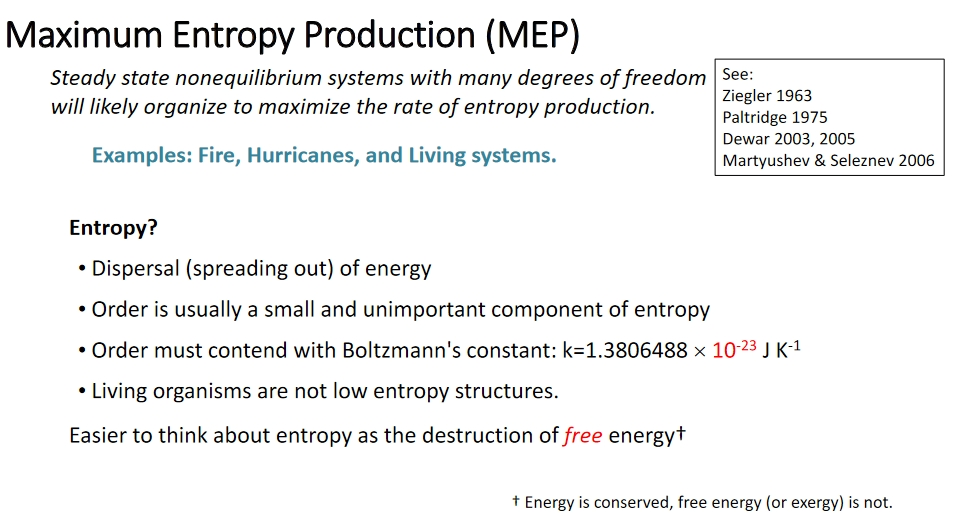Joe Vallino argues that living organisms are not especially low in entropy, since complex patterns don't count for much. He also argues that many systems organize themselves to maximize the rate of entropy production.

The first is just true; the second controversial.

As a mathematical physicist I'd like to derive the principle of maximum entropy production. As a biologist, Vallino uses it to study complex ecosystems.

Instead of modeling organisms (too many kinds), he focuses on the chemical reactions they cause.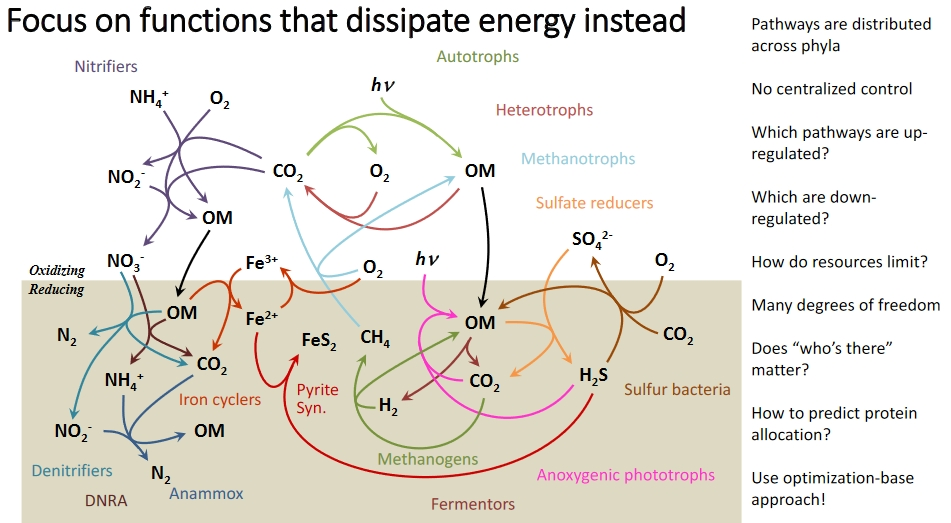I want to use network theory — and thus, categories — to study this stuff. But first I need to learn it better. So I'm running some conference sessions on it, like this:

You can see slides of Vallino's talk here:

#### June 17, 2021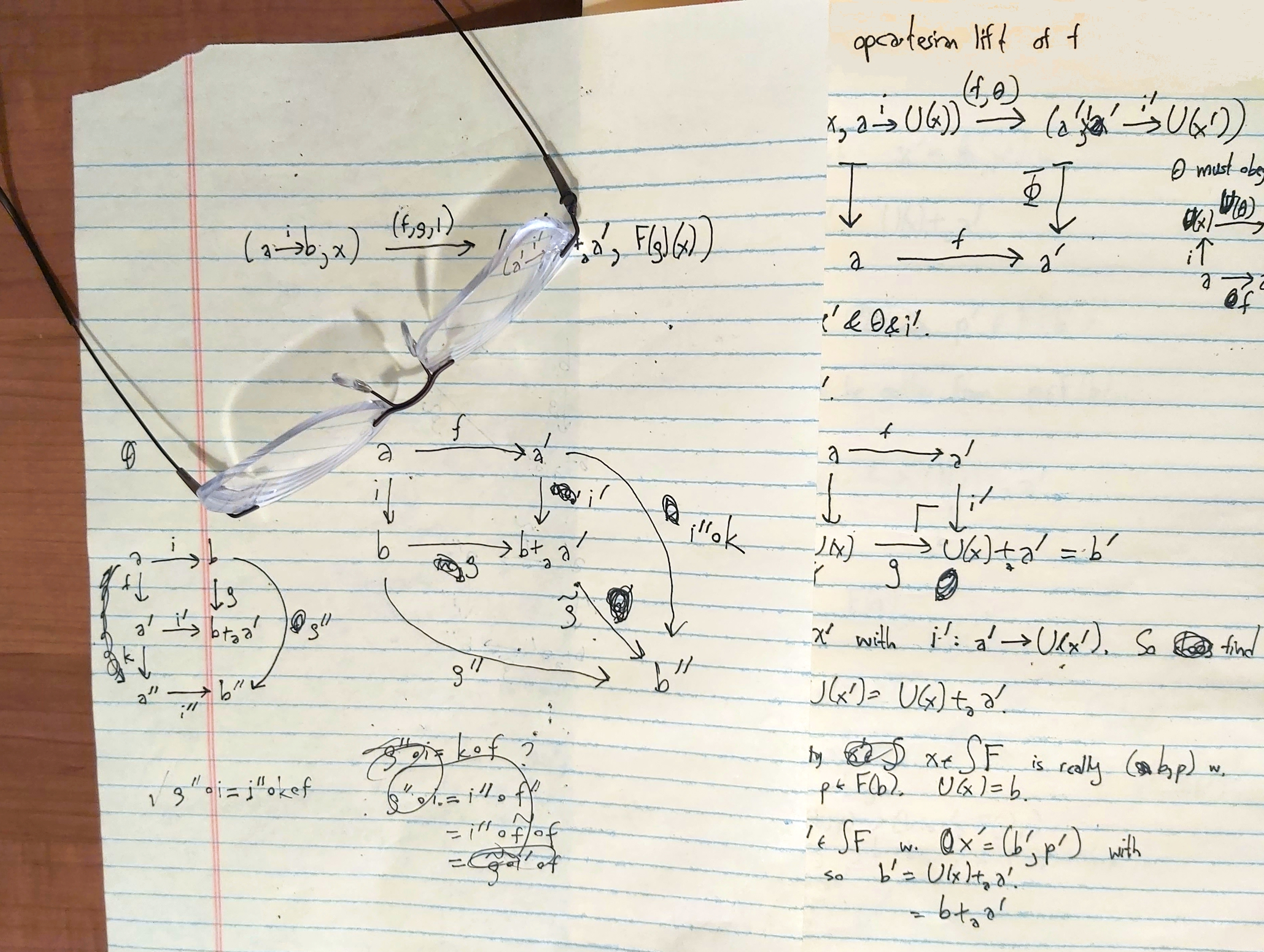Category theory when I'm thinking about it: results so beautiful they're obviously true.

Category theory when I'm writing about it: pages and pages of calculations full of mistakes.

I'm working to finish up my paper Structured versus decorated cospans with Kenny Courser and Christina Vasilakopoulou. We're massively improving the proofs of our main results using an idea suggested by Michael Shulman. After a long break spent working on other papers, it was a bit tough for me to get back up to speed on the details of this work. But since this is the last paper I need to finish with students before I retire, and I'm done teaching, I was able to spend whole days thinking about just this. And that's great! After uploading everything into my brain again, it became a lot easier to make rapid progress. Everything is falling into place beautifully.

#### June 18, 2021

An organism can only have a large genome if it has a low mutation rate. A tiny viroid can survive with one copy error per 500, but humans have DNA proofreading and repair mechanisms that bring the error rate down to one per 10 billion.

For more on this, check out these talk slides:

This is where I got the above figure. It's pretty cool stuff. Mathematicians should prove more theorems about these things! On Twitter, Rhonaldo wrote:
Martin Nowak (in his 2006 book, Evolutionary Dynamics) used a simple model to show that $$u L \lt 1$$ where $$u$$ is the mutation rate per base and $$L$$ is the genome length. He used the principle that adaptation is localization in the fitness landscape. Book worth checking out.

The model used to justify $$uL \lt 1$$ is in Chapter 3. He used the quasispecies equation and simplifying assumptions:

• the genome base type binary (0 or 1)
• two genomes (or species), the first a higher fitness wild type, the second, mutants.
• no back mutation from mutants to wild type.

#### June 20, 2021

You can put 5 tetrahedra in a dodecahedron in such a way that every rotational symmetry of the dodecahedron permutes these tetrahedra. In fact there are two ways to do this, a left-handed and a right-handed way! Here Greg Egan drew one way: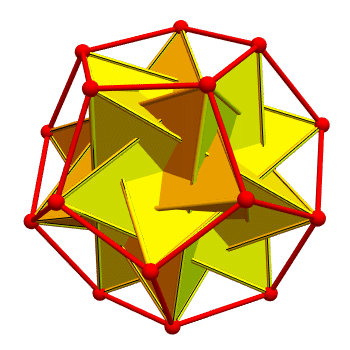From this you can see that the rotational symmetry group of the dodecahedron is a subgroup of $$\mathrm{S}_5$$, the group of permutations of 5 things. But $$\mathrm{S}_5$$ has 5! = 120 elements. Are all of these rotational symmetries of the dodecahedron?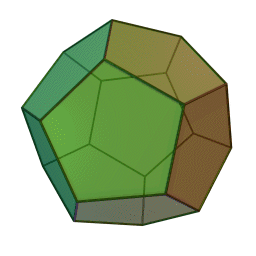Well, many rotational symmetries does the dodecahedron have? You can rotate it to carry any of its 12 pentagons to any other — but you can do that in 5 ways since you can rotate the pentagon. So, it has just

5 × 12 = 60
rotational symmetries.

60 is half of 120. So, the rotational symmetries of the dodecahedron form a subgroup of $$\mathrm{S}_5$$ containing just half the elements. An obvious guess is $$\mathrm{A}_5$$, the group of even permutations of the 5 tetrahedra.

And you can show that's true — either by checking, or by proving $$\mathrm{A}_5$$ is the only subgroup of $$\mathrm{S}_5$$ containing half the elements.

But a dodecahedron also has 6 'axes' connecting opposite faces.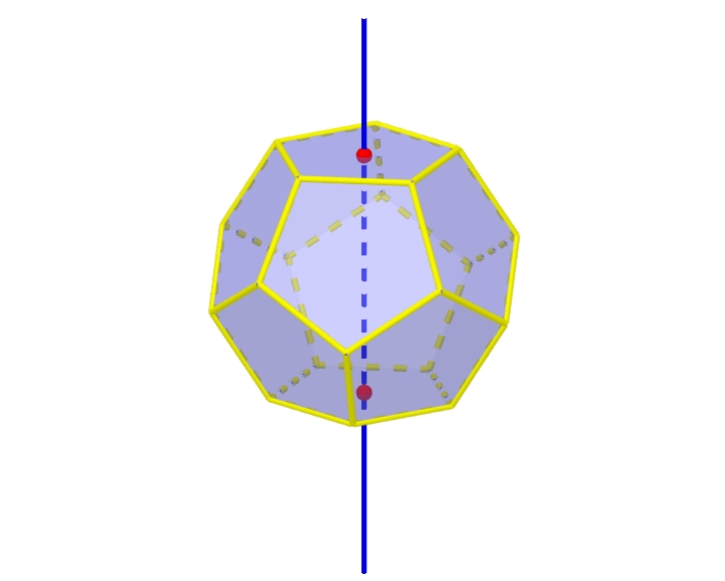So $$\mathrm{A}_5$$ has an interesting action on this 6-element set of axes. Indeed $$\mathrm{A}_5$$ acts transitively on the 6-element set of axes: in other words, you can rotate the dodecahedron to carry any axis to any other.

Can we get the larger group $$\mathrm{A}_5$$ to act on this 6-element set, in a way that extends how we've gotten $$\mathrm{6}_5$$ to act on it?

Yes we can! So we get something nice: permutations of a 5-element set acting transitively on a 6-element set. But it's not simple to explain — for me, anyway. I know a rather tricky geometrical description, and I know a couple of algebraic descriptions.

For example, the 6 axes of a dodecahedron correspond to the 6 five-element subgroups of $$\mathrm{A}_5$$. They're all cyclic groups and each one of them cyclically permutes 5 tetrahedra as shown below. You can see why we get a group like this for each axis.Since $$\mathrm{A}_5$$ acts to permute the 6 axes, it acts to permute these 6 five-element subgroups. And this action is just conjugation! In other words, if $$H$$ is one of these five-element subgroups, $$gHg^{-1}$$ will be another, for any $$g \in \mathrm{A}_5$$.

But these 6 five-element subgroups of $$\mathrm{A}_5$$ are also all the 5-element subgroups of $$\mathrm{S}_5$$. And $$\mathrm{S}_5$$ acts by conjugation to permute these subgroups!

So, our action of $$\mathrm{A}_5$$ on the 6 axes extends to an action of $$\mathrm{S}_5$$. But we're doing algebra now: the geometry is hidden.

There's a payoff, though. There are two ways for $$\mathrm{S}_5$$ to act on a 6-element set: the weird transitive action we just came up with, and the obvious action where it permutes 5 points and leaves the 6th alone. So, there are two kinds of $$\mathrm{S}_5$$ subgroups of $$\mathrm{S}_6$$.

And here's the kicker: there's an automorphism of $$\mathrm{S}_6$$ that switches the two kinds of $$\mathrm{S}_5$$. It maps the 'obvious' $$\mathrm{S}_5$$ subgroups to the 'weird' ones and vice versa!

This has to be an outer automorphism, since conjugation maps the 'obvious' $$\mathrm{S}_5$$ subgroups to 'obvious' ones.

What's really amazing is this: $$\mathrm{S}_6$$ is the only symmetric group that has any outer automorphisms. So the number 6 is special. And its specialness is connected to the dodecahedron, sporadic finite simple groups, and lots of other amazing things in math.

As you may have noticed, all this material is a watered-down version of my birthday present from David Harden, who pointed out that when I turned 60, I was also 6! months old — and thus deeply connected to both the dodecahedron's rotational symmetries and $$\mathrm{S}_6$$.

#### June 22, 2021The table-top mountains of Venezuela and Guyana are ecological islands, isolated from the forests below. They are remnants of a supercontinent over half a billion years old: Gondwana.

There are about 115 of these mountains, called tepui. The one here is Mount Roraima. It lies at the meeting point of Venezuela, Guyana and Brazil.

The world's tallest waterfall, Angel Falls, drops off the edge of another one of these tepui.

The tops of tepui are cool, with frequent rainfall. They have lots of carnivorous plants, due to depletion of nitrates over the ages. Some tepui have enough limestone that they have caves — and sinkholes formed when the roofs of tunnels carved by underground rivers collapse! These sinkholes are ecological 'islands within islands'.

#### June 24, 2021The Marimbalite was an electronic instrument you could play like a marimba — but using flashlights instead of mallets. It was invented in 1935 by an engineer at Westinghouse, but it never caught on.

Dozens of photo-cells and radio tubes are lined up side by side atop the new musical device. For each musical note there is an oscillating circuit which produces electrical vibrations when light is directed on that circuits photo-cell.This is just one of many strange instruments shown here:

The Clavecin Électrique, the Telharmonium, the Dynaphone, the Superpiano, the Rhythmicon, the Vibroexponator, the Terpsitone, the Melodium, and many more!

#### June 25, 2021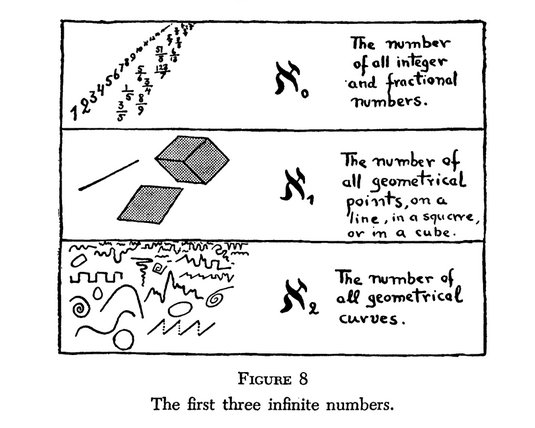As a kid I loved Gamow's book One Two Three... Infinity, and this picture. I felt ripped off when I learned that what he's calling $$\aleph_1$$ is really $$\beth_1$$.

Maybe it's not so bad: the Continuum Hypothesis says $$\aleph_1 = \beth_1$$. Maybe he's assuming that.

By definition $$\aleph_0 = \beth_0$$ is the smallest infinite cardinal. It's the cardinality of the set of integers, or rational numbers. But $$\aleph_1$$ is the smallest cardinal bigger than $$\aleph_0$$, while $$\beth_1$$ is defined to be $$2^{\aleph_1}$$. $$\beth_1$$ is the cardinality of the set of real numbers.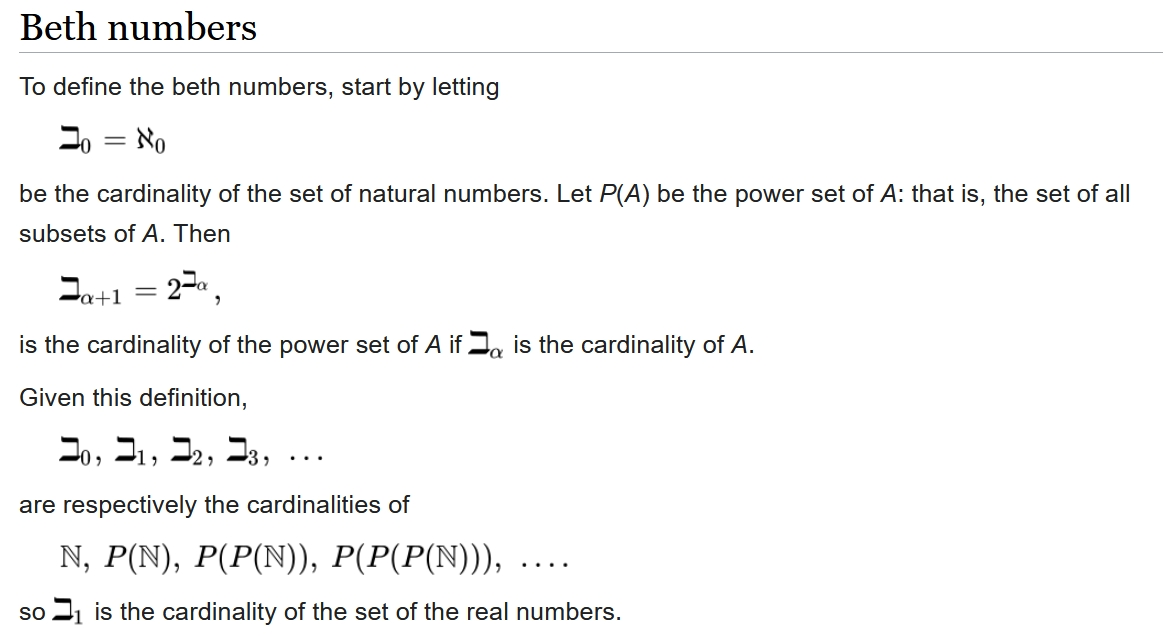Continuing on, we define $$\aleph_{n+1}$$ to be the smallest cardinal bigger than $$\aleph_n$$. We define $$\beth_{n+1}$$ to be $$2^{\beth_n}$$.

The Generalized Continuum Hypothesis says that $$\aleph_n = \beth_n$$ for all $$n$$. And not just all finite $$n$$: $$n$$ can be infinite. The index $$n$$ here is any 'ordinal'.

If $$n$$ is not some other ordinal plus one, we need to define $$\aleph_n$$ another way. We say it's the smallest cardinal bigger than all the $$\aleph_m$$ with $$m \lt n$$.

Similarly in this case $$\beth_n$$ is the smallest cardinal bigger than all the $$\beth_m$$ with $$m \lt n$$.

Maybe I forgive Gamow for using the aleph numbers $$\aleph_1$$ and $$\aleph_2$$ when he really meant the beth numbers $$\beth_1$$ and $$\beth_2$$. But I wish the beths got more than 1% as much publicity as the alephs.

Could Gamow have been playing yet another joke on his friend Bethe?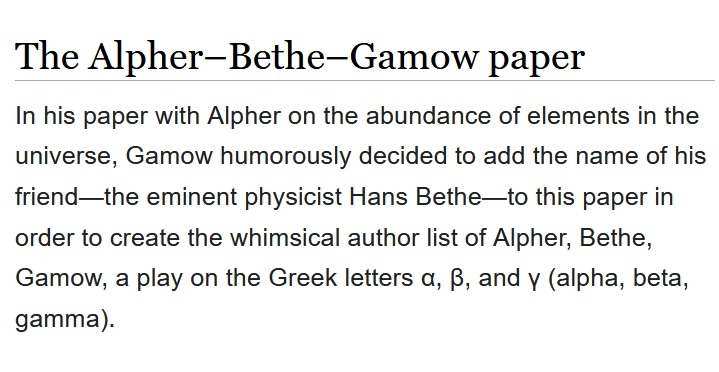#### June 26, 2021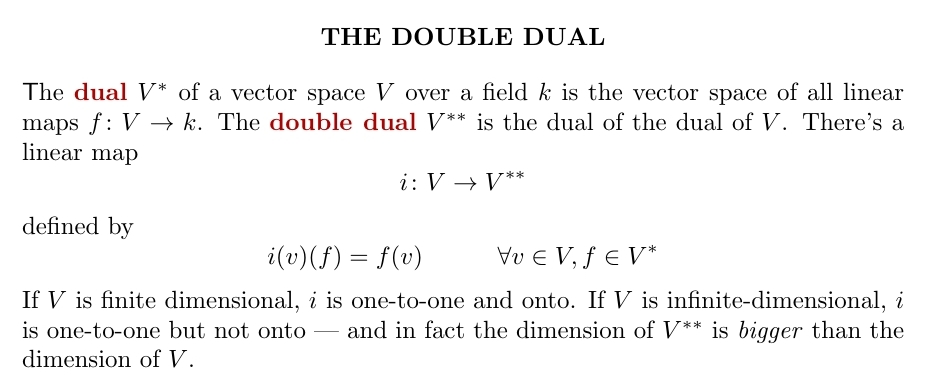The dual of a vector space $$V$$ is another vector space $$V^\ast$$. If $$V$$ is finite-dimensional, its dual has the same dimension. But if $$V$$ is infinite-dimensional, taking the dual always increases the dimension... so taking the dual twice increases it even more!

Since $$V$$ is included in $$V^{\ast \ast}$$, which is included in $$V^{\ast\ast\ast\ast}$$, and so on, we can build an enormous vector space by taking the union — or really 'colimit' — of all these!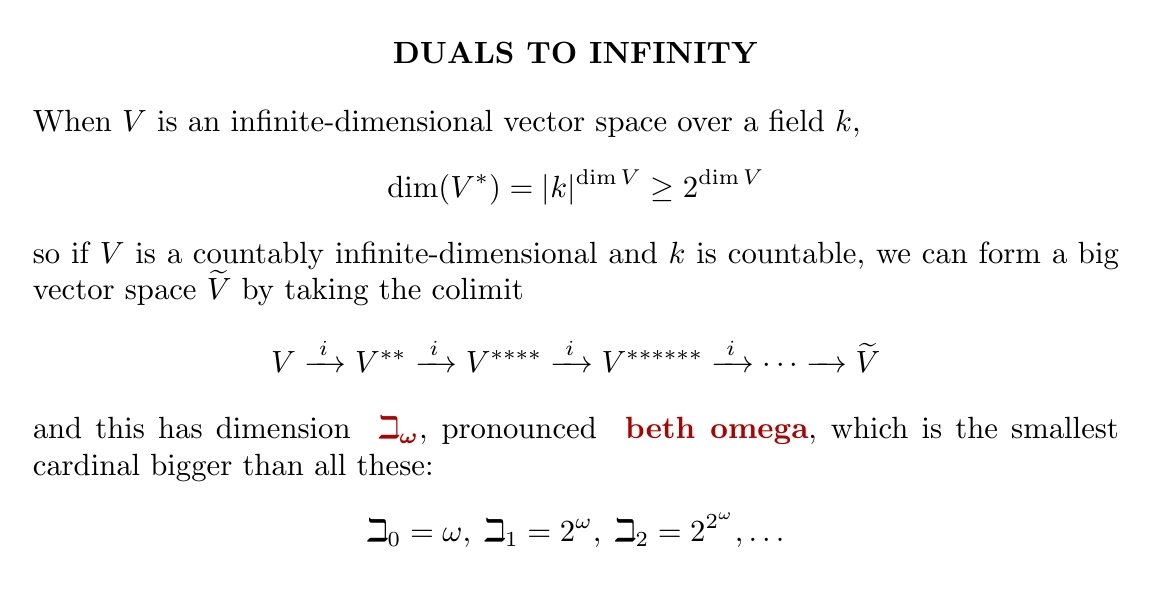When we start learning about infinity, we hear a lot about alephs — but a bit less about beths. For a great introduction to 'beths' read this:

• Tom Leinster, Large sets 6, The n-Category Café, June 24, 2021.

#### June 27, 2021

Recently I went to my office and picked up over a year's worth of mail. Among other things, I found this nice present from Pierre Martin-Dussaud:Indeed, the John Baezhedron is listed on Polytopia!

#### June 29, 2021

Complex analysis is usually very nice — but not always.

If a set of analytic functions takes at most countably many values at each point, is that set countable? Shockingly, this question can't be answered using the usual axioms of set theory!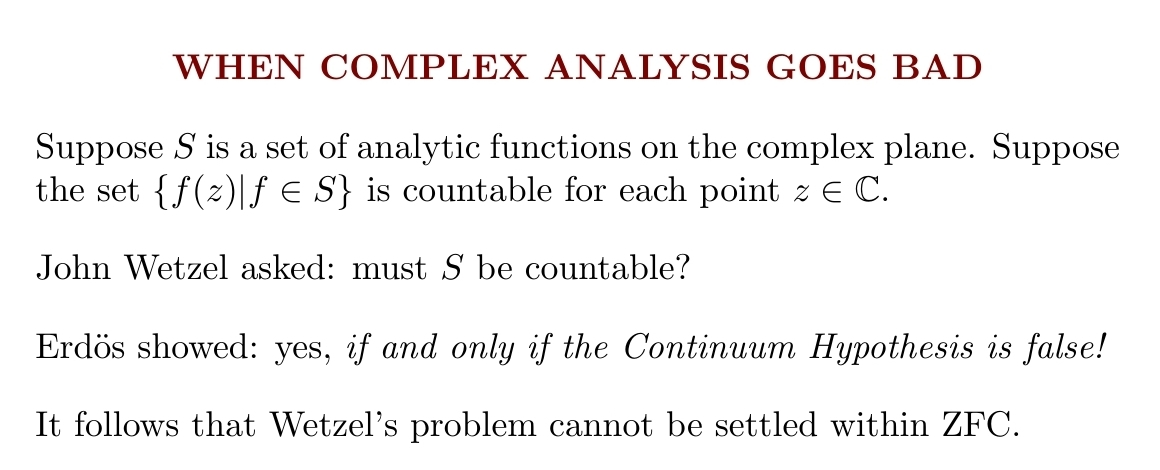I'd have guessed the answer is 'yes' — but Erdős showed that's true if and only if the Continuum Hypothesis is false!

One direction is super-easy: assume the cardinality of the continuum, c, is $$\aleph_1$$. Take $$\aleph_1$$ functions and find a point where they all have different values.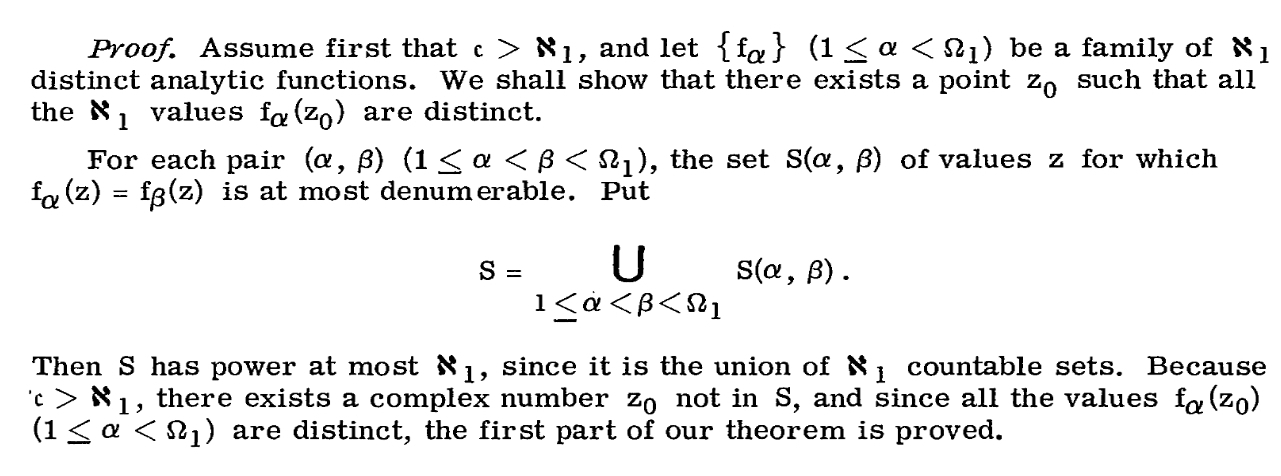Here Erdős 'lists' the functions using an ordinal $$\Omega_1$$ whose cardinality is $$\aleph_1$$. Note the set of values $$z$$ for which two distinct analytic functions can be equal is at most countable: otherwise these values would have a cluster point, so the functions would be equal.

So if the Continuum Hypothesis is false, given $$\aleph_1$$ distinct analytic functions we can find a point where they all take distinct values... ultimately because the plane has more than $$\aleph_1$$ points!

But what if it's true?

If the Continuum Hypothesis is true, Erdős can construct by induction an uncountable set of distinct analytic functions that take only countably many different values at each point in the plane.

The argument is here — it's fiendishly clever but doesn't use anything deep. He says a family of analytic functions has property P0 if for each $$z \in \mathbb{C}$$ the set of their values at $$z$$ is countable.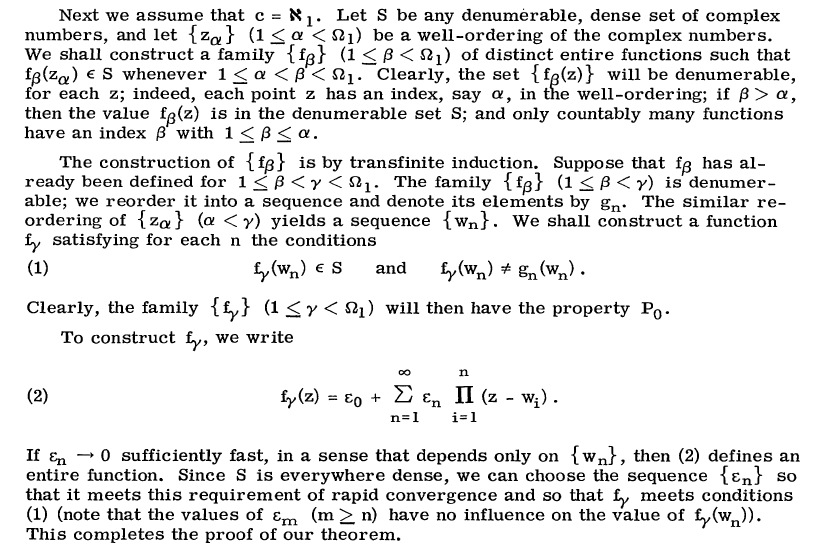Why does the surprising thing — an uncountable collection of distinct analytic functions taking only countably many distinct values at each point — happen when we assume the Continuum Hypothesis is true? This is important to understand.

The Continuum Hypothesis says we can well-order the real (or complex) numbers in such a way that each initial segment is countable. So, very roughly, they're "countable until we get to the very end". Erdős uses this shocking claim to get a shocking result.

Well... he also uses the Axiom of Choice to get this well-ordering, so you might blame that for his weird result. But maybe I can begin to see why Gödel and others believed the Continuum Hypothesis is 'false'. In a sense it asserts there are very few real numbers.

Erdős' paper is just 2 pages long, and it's open-access here:

For more of the history of Wetzel's problem, read this:

#### June 30, 2021

More progress:
1. Thursday June 8, 2021 - Speak at Categories and Companions 2021. DONE.
2. Monday June 14, 2021 - Larry Li, Bill Cannon and I run a session on "Non-equilibrium Models in Biology: from Chemical Reaction Networks to Natural Selection" at the annual meeting of the Society for Mathematical Biology DONE. I'm talking to Hong Qian about thermodynamics and biology now, and I want to spend more time on this stuff.
3. Wednesday June 23, 2021 - Talk at Google on The answer to the ultimate question of life, the universe and everything. DONE.
4. Finish Structured versus decorated cospans with Kenny Courser and Christina Vasilakopoulou. THIS IS THE MAIN THING I NEED TO DO. It looks done, and the new proofs based on an idea of Mike Shulman make the paper a lot nicer. I just need Kenny and Christina to agree it's ready for me to put it on the arXiv.
5. Thursday July 1, 2021 - I retire and drive to Berkeley to visit the Topos Institute.